# Surface Areas And Volumes Solution of TS & AP Board Class 9 Mathematics

#### Surface Areas And Volumes Solution of TS & AP Board Class 9 Mathematics

###### Exercise 10.1

Question 1.

Find the later surface area and total surface area of the following right prisms.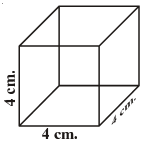Given, l = b = h = 4cm.

Formula Used:- total surface area = 2(lb + bh + lh)

Lateral surface area = 2h(l + b)

Here all dimensions are equal i.e. l = b = h = a(say)

Hence, total surface area = 6a2

= 6 × 42

= 96cm2

Lateral surface area = 4a2

= 4 × 42

= 64cm2

Question 2.

Find the later surface area and total surface area of the following right prisms.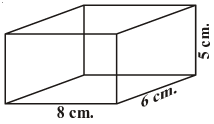Given, length(l) = 8cm

Breath(b) = 6cm

Height(h) = 5cm

Formula Used:- total surface area = 2(lb + bh + lh)

Lateral surface area = 2h(l + b)

Hence, total surface area = 2((8 × 6) + (6 × 5) + (5 × 8))

= 236cm2

Lateral surface area = (2 × 5)(8 + 6)

= 10 × 14

= 140cm2

Question 3.

The total surface area of a cube is 1350 sq.m. Find its volume.

Given, total surface area of a cube = 1350m2

Let side of the cube be ‘a’

Formula used:- volume of cube = a3

Total surface area = 6a2

Hence, 6a2 = 1350

a2 =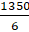a =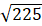a = 15m

volume of the cube = a3

= 153

= 3375m3

Question 4.

Find the area of four walls of a room (Assume that there are no doors or windows) if its length 12 m., breadth 10 m. and height 7.5 m.

Given, length(l) = 12m

Breath(b) = 10m

Height(h) = 7.5m

We know, in case of area of four wall which is formed a cuboid, eliminate the area of base and top i.e. (l × b) Hence,

Area of four walls = 2(bh + lh)

= 2((10 × 7.5) + (12 × 7.5))

= 2(165)

= 330m2

Question 5.

The volume of a cuboid is 1200 cm3. The length is 15 cm. and breadth is 10 cm. Find its height.

Given, volume of a cuboid(v) = 1200cm3

Length(l) = 15cm

Breath(b) = 10cm

Volume of a cuboid = l × b × h

Hence, l × b × h = 1200

15 × 10 × h = 1200

h =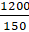h = 8cm

Question 6.

How does the total surface area of a box change if

(i) Each dimension is doubled?

(ii) Each dimension is tripled?

Express in words. Can you find the area if each dimension is raised to n times?

(i) If each dimension is doubled then the total surface area becomes, = 2((4lb) + (4bh) + (4lh))

= 4 × [2(lb + bh + lh)]

∴ the area becomes four times.

(ii) If each dimension is tripled then the total surface area becomes, = 2((9lb) + (9bh) + (9lh))

= 9 × [2(lb + bh + lh)]

∴ the area becomes nine times.

It is clear from the above two solutions that the area of cuboid becomes n2times the previous area if each dimension raised to n times.

Question 7.

The base of a prism is triangular in shape with sides 3 cm., 4 cm. and 5 cm. Find the volume of the prism if its height is 10 cm.

Given, a triangular prism with base dimensions 3cm, 4cm and 5cm. and height is 10cm

Volume of this type of prism = area of base × height

The triangle is right angled triangle. Hence, area of base is× 4 × 3.

Volume =× 4 × 3 × 10

= 60cm3

Question 8.

A regular square pyramid is 3 m. height and the perimeter of its base is 16 m. Find the volume of the pyramid.

Given, A regular square pyramid with base 16m and height is 3m.

Volume of the pyramid =× area of the base × height× 162 × 3

= 256m3

Question 9.

An Olympic swimming pool is in the shape of a cuboid of dimensions 50 m. long and 25 m. wide. If it is 3 m. deep throughout, how many liters of water does it hold?

Given, An Olympic swimming pool is in the shape of a cuboid of dimensions 50 m. long and 25 m. wide and 3m deep.

For calculating the volume of water required into pool, we need to find the volume of cuboidal pool i.e. (lbh).

Volume = l × b × h

= 50 × 25 × 3

= 3750m3

###### Exercise 10.2

Question 1.

A closed cylindrical tank of height 1.4 m. and radius of the base is 56 cm. is made up of a thick metal sheet. How much metal sheet is required (Express in square meters)

Given, radius of the base of a cylinder r = 56cm = 0.56m

And height h = 1.4m

To find the metal sheet required, we need to find the total surface area of the cylinder.

Total surface area of the cylinder = 2πr(r + h)

= 2 × π × 0.56 × (0.56 + 1.4)

= 6.89 ≃ 6.9m2

Question 2.

The volume of a cylinder is 308 cm3. Its height is 8 cm. Find its later surface area and total surface area.

Given, volume of cylinder = 308 cm3

Height = 8cm

Volume of the cylinder = πr2h

308 = 3.14 × r2 × 8

r2 =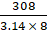r =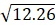r = 3.5cm

lateral surface area = 2πrh

= 2 × 3.14 × 3.5 × 8

= 175.9≃ 176cm2

Total surface area = 2πr(r + h)

= 2 × π × 3.5 × (3.5 + 8)

= 252.89 ≃ 253cm2

Question 3.

A metal cuboid of dimension 22 cm. × 15 cm. × 7.5 cm. was melted and cast into a cylinder of height 14 cm. What is its radius?

Given, A metal cuboid of dimension 22 cm. × 15 cm. × 7.5 cm. was melted and cast into a cylinder of height 14 cm

According to the question the volume remains same after casting the cuboid inti cylinder, i.e. lbh = πr2h.

22 × 15 × 7.5 = 3.14 × r2 × 14

r2 =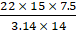r =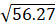r = 7.5cm

Question 4.

An overhead water tanker is in the shape of a cylinder has capacity of 616 litres. The diameter of the tank is 5.6 m. Find the height of the tank.

Given, An overhead water tanker is in the shape of a cylinder has capacity of 616 litres

As 1 litre = 0.001 m3

the volume of the cylinder = 0.616 m3.

Diameter of the cylinder = 5.6 m

Radius of the cylinder =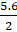= 2.8 m

Volume of the cylinder = πr2h.

0.616 = 3.14 × 2.82 × h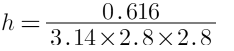Question 5.

A metal pipe is 77 cm. long. The inner diameter of a cross section is 4 cm., the outer diameter being 4.4 cm. (see figure) Find its

(i) inner curved surface area

(ii) outer curved surface area

(iii) Total surface area.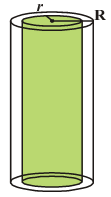Given, A metal pipe is 77 cm. long

Inner Diameter = 4cm i.e. r = 2cm

Outer diameter = 4.4cm i.e. R = 2.2cm

(i) inner curved surface area = 2πrh

= 2 × 3.14 × 2 × 77

= 967.6cm2

(ii) outer curved surface area = 2πRh

= 2 × 3.14 × 2.2 × 77

= 1063.8cm2

(iii) total surface area = 2πrh + 2πrh + 2π(R2 – r2)

= 967.6 + 1063.8 + 2 × 3.14 × (2.22 – 22)

= 2036.6cm2

Question 6.

A cylindrical piller has a diameter of 56 cm and is of 35 m high. There are 16 pillars around the building. Find the cost of painting the curved surface area of all the pillars at the rate of 5.50 per 1 m2.

Given, A cylindrical pillar, diameter = 56cm = 0.56m i.e. r = 0.28cm

Height h = 35m

Number of pillars n = 16

Curved surface area of a pillar = 2πrh

= 2 × 3.14 × 0.28 × 35

= 61.54cmCurved surface area of 16 pillars = 16 × 61.54

= 984.64cm2

Cost of painting = 5.5 × 984.64

= 5415.52

Question 7.

The diameter of a roller is 84 cm and its length is 120 cm. It takes 500 complete revolutions to roll once over the play ground to level. Find the area of the play ground in m2.

Given, diameter = 84cm = 0.84m i.e r = 0.42m

Length h = 120cm = 1.20m

Number of revolutions n = 500

Area of the playground = n × curved surface area of the roller

= 500 × 2πrh

= 500 × 2 × 3.14 × 0.42 × 1.2

= 1582.56m2

Question 8.

The inner diameter of a circular well is 3.5 m. It is 10 m deep. Find

(i) its inner curved surface area

(ii) The cost of plastering this curved surface at the rate of Rs. 40 per m2.

Given, inner diameter of a circular well = 3.5 m i.e. r = 1.75m

Height = 10m

(i) curved surface area = 2πrh

= 2 × 3.14 × 1.75 × 10

= 109.9m2≃ 110cm2

(ii) cost of plastering = 40 × 110

= 4400

Question 9.

Find

(i) The total surface area of a closed cylindrical petrol storage tank whose diameter 4.2 m. and height 4.5 m.

(i) Given, diameter = 4.2m

Height = 4.5m

Total surface area = 2πr(r + h)

= 2 × 3.14 × 2.1 × (2.1 + 4.5)

= 87m2

(ii) if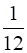of the steel was wasted in making the tank. The sheet used in making the tank = (1−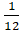) × 87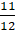× 87

= 79.75m2

Question 10.

A one side open cylinderical drum has inner radius 28 cm. and height 2.1 m. How much water you can store in the drum. Express in litres. (1 litre = 1000 cc.)

Given, r = 28cm

h = 2.1m = 210cm

to find the capacity of water ,the volume of the cylinder = πr2h

= 3.14 × 282 × 210

= 516969.6cm3

1litere = 1000cm3

Hence, the water stored =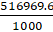= 516.9literes

Question 11.

The curved surface area of the cylinder is 1760 cm.2 and its volume is 12320 cm3. Find its height.

Given, curved surface area = 1760cm2

Volume = 12320cm3

For finding the height of the cylinder,

curved surface area = 1760cm2

2πrh = 1760

rh =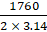rh = 280.25 --------(i)

volume = 12320cm3

πr2h = 12320cm3

r × rh =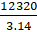r =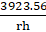from equation (i), r =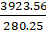r = 14cm

hence, rh = 280.25

h =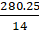h = 20cm

## MATHS VIDEOS